# Odd abundant number facts for kids

Kids Encyclopedia Facts

An odd abundant number is an odd number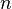$n$ letting its sum-of divisors greater than the twice of itself.

## Examples

• The first example is 945 (33× 5× 7) and having the abundance of 30. And the next twelve are
1575, 2205, 2835, 3465, 4095, 4725, 5355, 5775, 5985, 6435, 6615.
• Odd abundant numbers below 500000 are in On-Line Encyclopedia of Integer Sequences A005231.

## Formula

The formula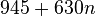$945+630n$

gives 62 odd abundant numbers, but fails when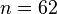$n=62$.

The second formula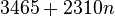$3465+2310n$

gives 192 abundant numbers, but fails when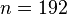$n=192$

Furthermore, the last formula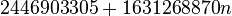$2446903305+1631268870n$

are abundant numbers, until , it appears to be the first counterexample.

## Special Properties

• An abundant twin is a pair of (n, n+1) and they are both abundant numbers. Abundant twins are usually less common. The first three pairs of abundant twins are (5984, 5985) (11024,11025) (81080, 81081).
• An calculation was given by Iannucci shows how to find the smallest abundant number not divisible by the first n primes.
• An abundant number with abundance 1 is called a quasiperfect number, although none have yet been found. A quasiperfect number must be an odd square number having a value above 1030

### n-rough

A n-rough abundant number is an abundant number not divisible by primes under$n$. In other words, they are abundant numbers that do not divide any integer from 2 to n.

n first n-rough abundant number Factorization
2 12
3 945
5 5391411025
7 20169691...76756331
11 112 × 132 × 17 × 19 × 23 × ... × 103 × 107 × 109 × 113 × 127 × 131 × 137
13 132 × 172 × 19 × 23 × ... × 223 × 227
17 172 × 192 × 232 × 29 × 31 × ... × 347 × 349
19 192 × 232 × 292 × 31 × 37 × ... × 491 × 499Odd abundant number Facts for Kids. Kiddle Encyclopedia.